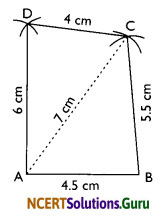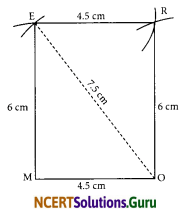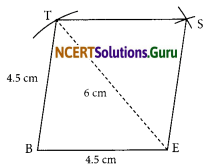# NCERT Solutions for Class 8 Maths Chapter 4 Practical Geometry Ex 4.1

These NCERT Solutions for Class 8 Maths Chapter 4 Practical Geometry Ex 4.1 Questions and Answers are prepared by our highly skilled subject experts.

## NCERT Solutions for Class 8 Maths Chapter 4 Practical Geometry Exercise 4.1

Question 1.
AB = 4.5 cm
BC = 5.5 cm
CD = 4 cm
AC = 7 cm

JU = 3.5 cm
UM = 4 cm
MP = 5 cm
PJ = 4.5 cm
PU = 6.5 cm(iii) Parallelogram MORE
OR = 6 cm
RE = 4.5 cm
EO = 7.5 cm

(iv) Rhombus BEST
BE = 4.5 cm
ET = 6 cm
Solution:
(i)Steps of Construction:
I. Draw a line segment AB = 4.5 cm.
II. With centre at A and radius = 7 cm, draw an arc.
III. With centre at B and radius = 5.5 cm, draw another arc to intersect the previous arc at C.
IV. With centre at A and radius = 6 cm, draw an arc on the side opposite to that of B.
V. With centre at C and radius = 4 cm, draw another arc to intersect the previous arc at D.
VI. Join AC, BC, DA, and DC. Thus, ABCD is the required quadrilateral.(ii)Steps of Construction:
I. Draw a line segment JU = 3.5 cm.
II. With centre at U and radius UP = 6.5 cm, draw an arc.
III. With centre at J and radius = 4.5 cm, draw another arc to intersect the previous arc at P.
IV. With centre at U and radius = 4 cm, draw an arc on the side opposite to that of J.
V. With centre at P and radius = 4 cm, draw another arc intersecting the previous arc at M.
VI. Join PJ, PU, PM, and UM. Thus, JUMP is the required quadrilateral.

(iii)Note: In a parallelogram opposite sides are equal.
Steps of Construction:
I. Draw a line segment MO = 4.5 cm
II. With centre O and radius = 7.5 cm, draw an arc.
III. With centre M and radius = 6 cm, draw another arc to intersect the previous arc at E.
IV. With centre at O and radius = 6 cm, draw an arc on the side opposite to E
V. With centre at E and radius = 4.5 cm, draw another arc to intersect the previous arc at R.
VI. Join OR, RE, EO, and EM. Thus, MORE is the required parallelogram.(iv)Steps of constructions:
I. Draw a line segment BE = 4.5 cm.
II. With centre at E and radius = 6 cm, draw an arc.
III. With centre at B and radius = 4.5 cm, draw another arc to intersect the previous arc at T.
Note: All 4 sides of a rhombus are equal.
IV. Join TB and TE.
V. With centre at T and radius = 4.5 cm, draw an arc on the side opposite to that of B.
VI. With centre at E and radius = 4.5 cm, draw another arc to intersect the previous arc at S.
VII. Join ST and SE. Thus, BEST is the required rhombus.

error: Content is protected !!